integration must be added to each integration. Second, the result of integration is a function of a variable and has no definite value, even after the constant of integration is determined, until the variable is asigned a numerical value."> Definite IntegralsCustom SearchDEFINITE INTEGRALS The general form of the indefinite integral is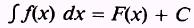and has two identifying characteristics. First, the constant of integration must be added to each integration. Second, the result of integration is a function of a variable and has no definite value, even after the constant of integration is determined, until the variable is asigned a numerical value. The definite integral eliminates these two characteristics. The form of the definite integral iswhere a and b are given values. Notice that the constant of integration does not appear in the final expression of equation (6.6). In words, this equation states that the difference of the values of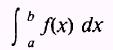for x=a and x=b gives the area under the curve defined by f(x), the X axis, and the ordinates where x=a and x=b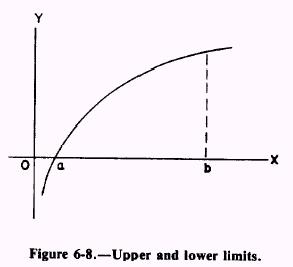In figure 6-8, the value of b is the upper limit and the value of a is the lower limit. These upper and lower limits may be any assigned values in the range of the curve. The upper limit is positive with respect to the lower limit in that it is located to the right (positive in our case) of the lower limit. Equation (6.6) is the limit of the sum of all the strips between a and b, having areas of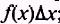; that is,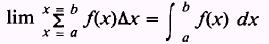The definite integral evaluated from a to b isThe notation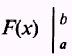in equation (6.7) means we first substitute the upper limit, b, into the function F(x) to obtain F(b); and from F(b) we subtract F(a), the value obtained by substituting the lower limit, a, into F(x). EXAMPLE: Find the area bounded by the curve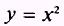the X axis, and the ordinates where x=2 and x=3 as shown in figure 6-9.SOLUTION: Substituting into equation (6.7), we have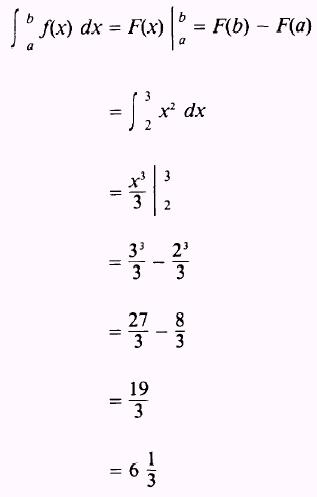We may make an estimate of this solution by considering the area desired in figure 6-9 as being a right triangle resting on a rectangle. The triangle has an approximate area of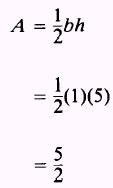and the area of the rectangle is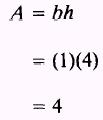so that the total area iswhich is a close approximation of the area found by the process of integration. EXAMPLE: Find the area bounded by the curve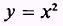the X axis, and the ordinates where x= -2 and x=2 as shown in figure 6-10.Figure 6-10.-Area under a curve.Integrated Publishing, Inc. - A (SDVOSB) Service Disabled Veteran Owned Small Business